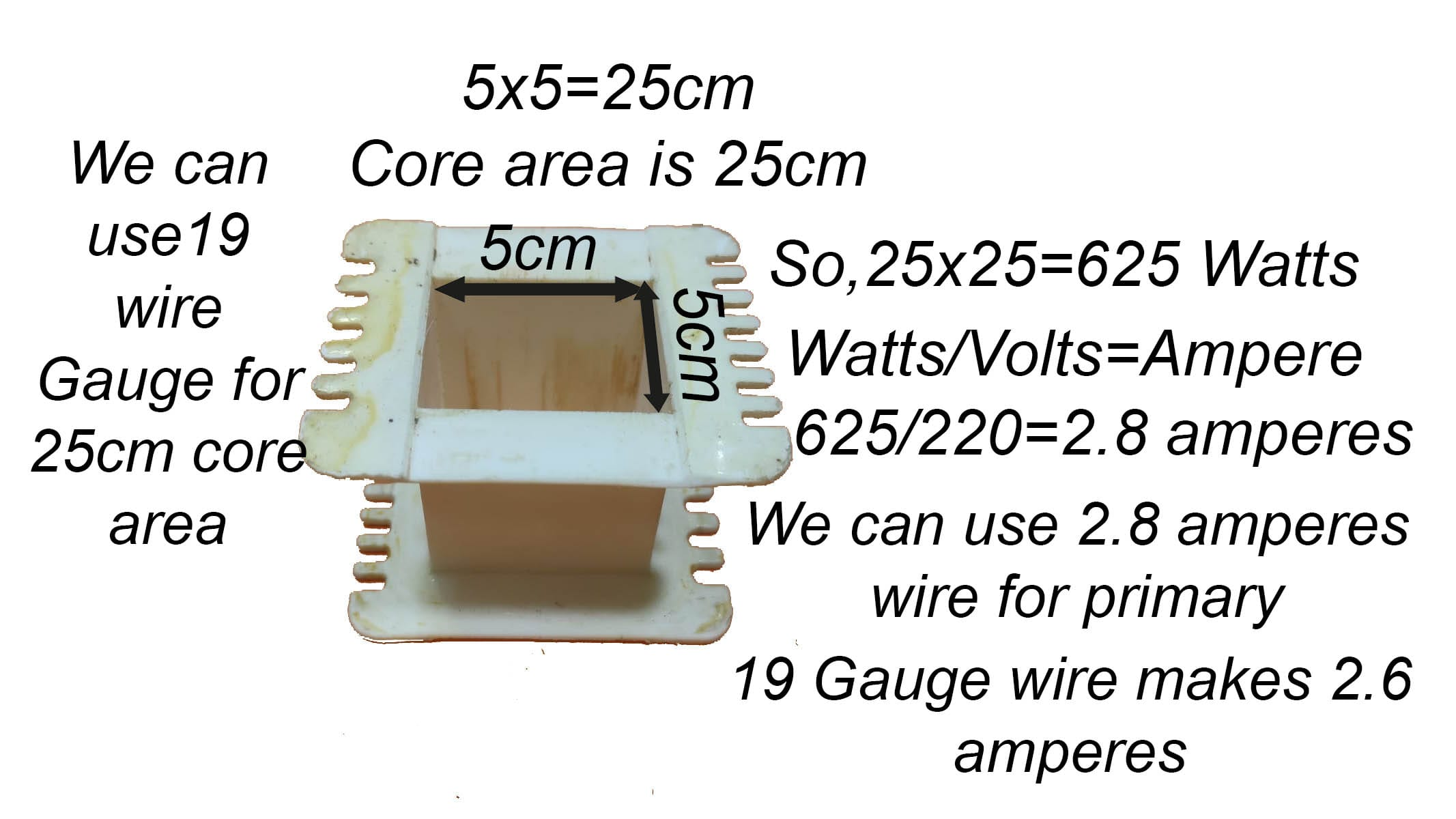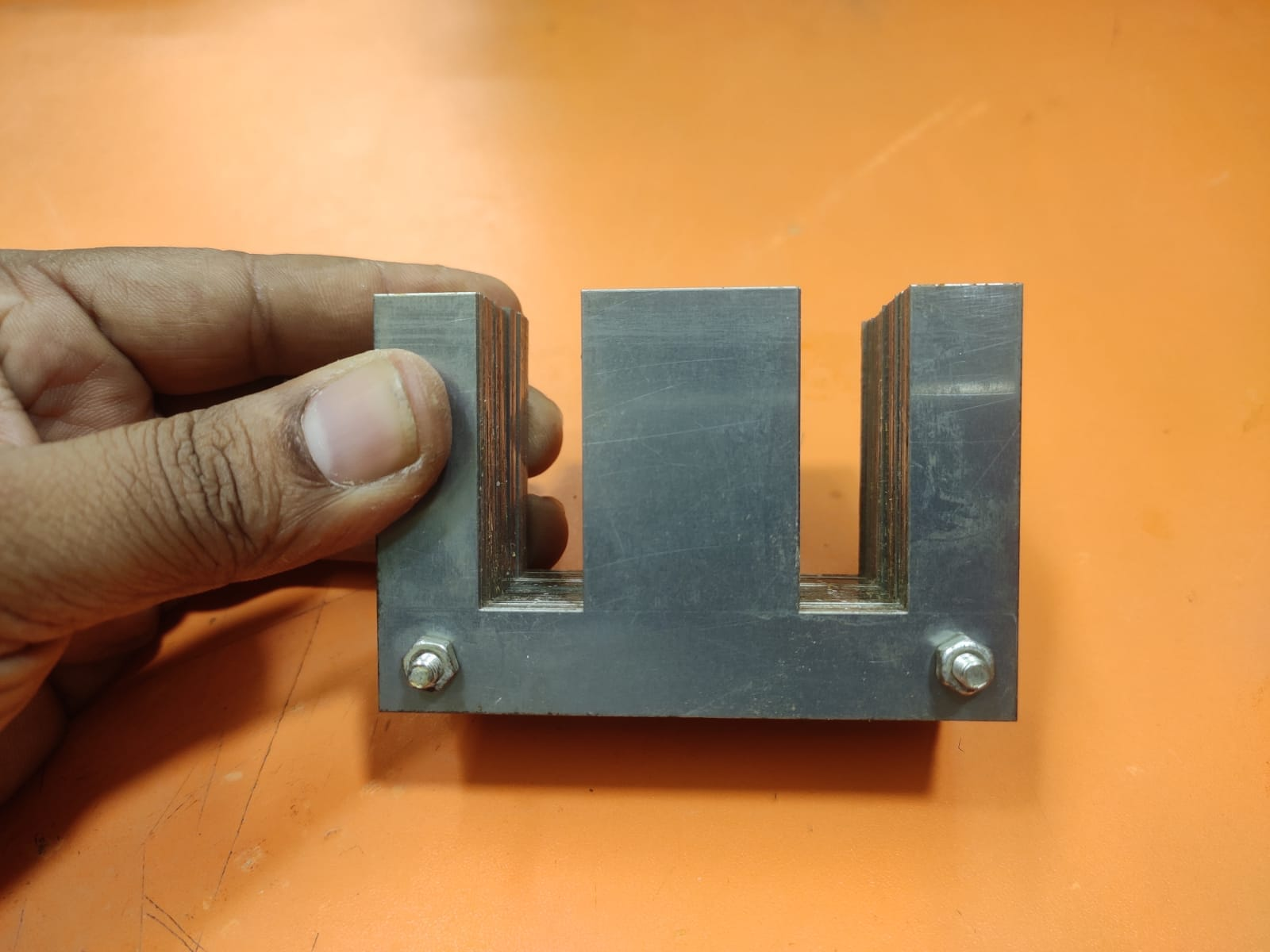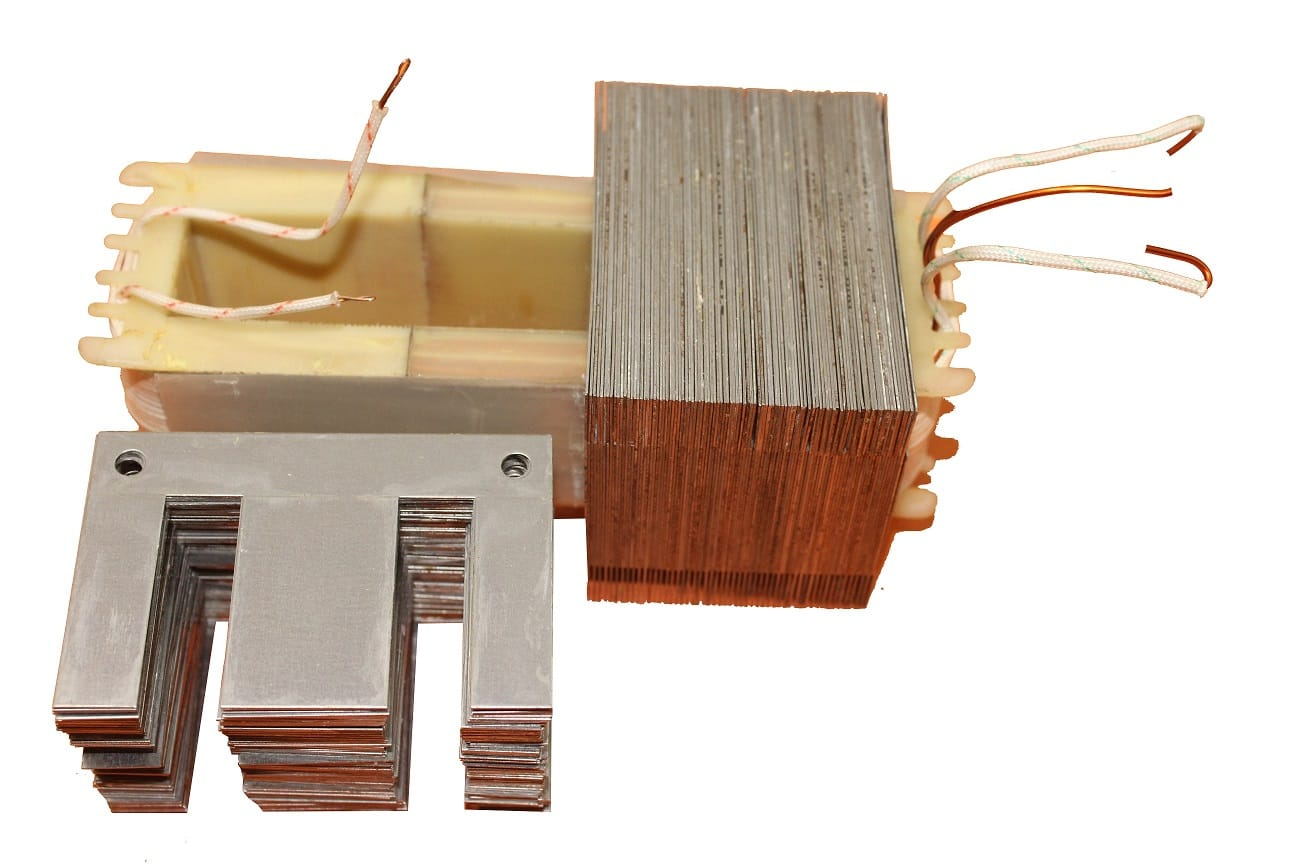# How to calculate wire gauge for transformer

## How to calculate the wire gauge for the transformer:

How to calculate the wire gauge for the transformer? transformer winding has rules. rules are the same for every size of the transformer. it depends on the core area. we have to know the core area first to choose the wire gauge. we have to choose wire according to the transformer watts.Here in this picture, we can see the transformer core area is 25cm. So, 25×25=625 is the transformer watts. this transformer can make a maximum of 625 watts. and we also know that watts/volt=amperes.

watts is 625 and the Ac voltage is 220. so, 625/220=2.8 ampres. for this transformer, we can use 19 number wire gauge.This is the E core for this transformerThanks a lot to be with us. If you want another post then please visit our website.

We have another post for you. like a Bass circuit diagram

Please like comment and share our post.